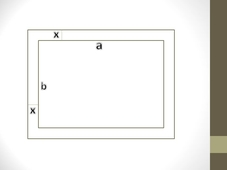# A 3-inch by 5-inch picture is put into a frame with a mat that has width x. Draw picture inside...

## Question:

A 3-inch by 5-inch picture is put into a frame with a mat that has width x. Draw picture inside the frame, write the expression of the width and length of the frame and the picture together. If the total area of the frame with the picture is {eq}35 in^2 {/eq}, find the width and length of the frame.

## Area of a Rectangle shape:

In geometry, a rectangle is a planner or we can say two-dimensional shape that is known for having a pair of parallel sides that are also equal in length.

Each angle is a right angle in a rectangle so it is a parallelogram.

#### Area of Rectangle

The area of the rectangle is the amount of space enclosed by it on two dimensional or planner space.

Formula-

{eq}\displaystyle A = a \times b {/eq}

here a and b are the length and width of the rectangle

A is the area of the rectangle

#### Perimeter of the Rectangle

It is given by the sum of all sides of a rectangle

Formula-

{eq}\displaystyle P = 2(a+b) {/eq}

here P is the perimeter of the rectangle

Given that a 3-inch by 5-inch picture is put into a frame with a mat that has a width x.

{eq}\displaystyle a = 3 ~inch {/eq}

{eq}\displaystyle b = 5 ~inch {/eq}

So the area of the picture-

{eq}\displaystyle A = (3)(5) = 15 ~inch^{2} {/eq}

{eq}\displaystyle ab = 15 -------(1) {/eq}

So the corresponding figure is-So the new dimensions of the frame and picture together-

length {eq}\displaystyle (a') = a+2x {/eq}

{eq}\displaystyle a' = 3+2x {/eq}

width {eq}\displaystyle (b') = b+2x {/eq}

{eq}\displaystyle b' = 5+2x {/eq}

Also given that the total area of the frame with the picture is {eq}\displaystyle 35~ inch^{2}. {/eq}

{eq}\displaystyle A' = 35 ~inch^{2} {/eq}

{eq}\displaystyle (3+2x)(5+2x) = 35 {/eq}

{eq}\displaystyle 15+16x+4x^{2} -35 = 0 {/eq}

{eq}\displaystyle 4x^{2}+16x-20 = 0 {/eq}

{eq}\displaystyle x^{2}+4x-5 = 0 {/eq}

by factoring

{eq}\displaystyle x^{2}+5x-x-5 = 0 {/eq}

{eq}\displaystyle x(x+5)-1(x+5) = 0 {/eq}

{eq}\displaystyle (x+5)(x-1) = 0 {/eq}

x is the width of the frame so it can be negative.

{eq}\displaystyle x - 1 =0 {/eq}

{eq}\displaystyle x = 1~ inch {/eq}

{eq}\displaystyle a' = 3+2(1) = 5 ~inches {/eq}

{eq}\displaystyle b' = 5+2(1) = 7 ~inches {/eq}

So the length and width of the frame are 5 and 7 inches respectively.